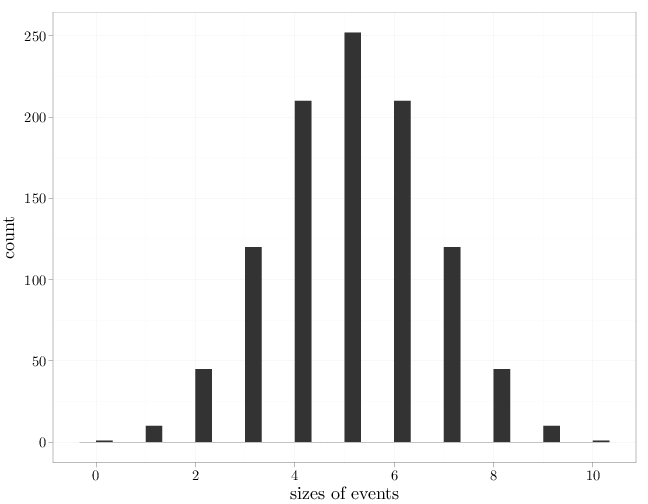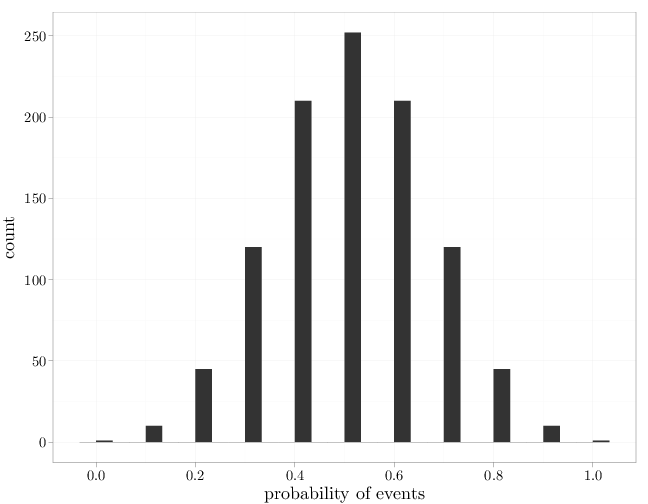## Probability

### The Analysis of Data, volume 1

Basic Definitions: The Probability Functions

## 1.3. The Classical Probability Model on Finite Spaces

In the classical interpretation of probability on finite sample spaces, the probabilities of all elementary events $\{\omega\}, \omega \in \Omega$, are equal. Since the probability function must satisfy $\P(\Omega)=1$ we have $\P(\{\omega\})=|\Omega|^{-1}, \qquad \text{for all}\quad \omega\in\Omega.$ This implies that under the classical model on a finite $\Omega$, we have $\P(E)=\frac{|E|}{|\Omega|}.$

Example 1.3.1. Consider the experiment of throwing two distinct dice and observing the two faces with order. The sample space is $\Omega=\{1,\ldots,6\}\times \{1,\ldots,6\}=\{(x,y):x,y\in\{1,2,\ldots,6\}\}$ (see Chapter A in the appendix for the notation of a Cartesian product of two sets). Since $\Omega$ has 36 elements, the probability of the elementary event $E=\{(4,4)\}$ is $\P(E)=1/|\Omega|=1/36$. The probability of getting a sum of 9 in both dice is \begin{align*} \P(\text{sum}=9) &= \P(\{(6,3),(3,6),(4,5),(5,4)\}) \\ &=\frac{|\{(6,3),(3,6),(4,5),(5,4)\}|}{36} \\ &= \frac{4}{36}. \end{align*} The classical model in this case is reasonable, assuming the dice are thrown independently and are fair.

The R code below demonstrates the classical model and the resulting probabilities on a small $\Omega$.

Omega = set(1, 2, 3)
# all possible events
2^Omega

## {{}, {1}, {2}, {3}, {1, 2}, {1, 3}, {2, 3},
##  {1, 2, 3}}

# size of all possible events
sapply(2^Omega, length)

##  0 1 1 1 2 2 2 3

# probabilities of all possible events under the
# classical model
sapply(2^Omega, length)/length(Omega)

##  0.0000 0.3333 0.3333 0.3333 0.6667 0.6667
##  0.6667 1.0000


Note that the sequence of probabilities above does not sum to one since it contains probabilities of non-disjoint events. The R code below demonstrates this below for a larger set using by graphing the histogram of sizes and probabilities.

library(ggplot2)
Omega = set(1, 2, 3, 4, 5, 6, 7, 8, 9, 10)
# histogram of sizes of all possible events
qplot(sapply(2^Omega, length), xlab = "sizes of events")# histogram of probabilities of all possible
# events under classical model
qplot(sapply(2^Omega, length)/length(Omega), xlab = "probability of events")The left-most and right-most bars represent two sets with probabilities 0 and 1, respectively. These sets are obviously $\emptyset$ and $\Omega$.